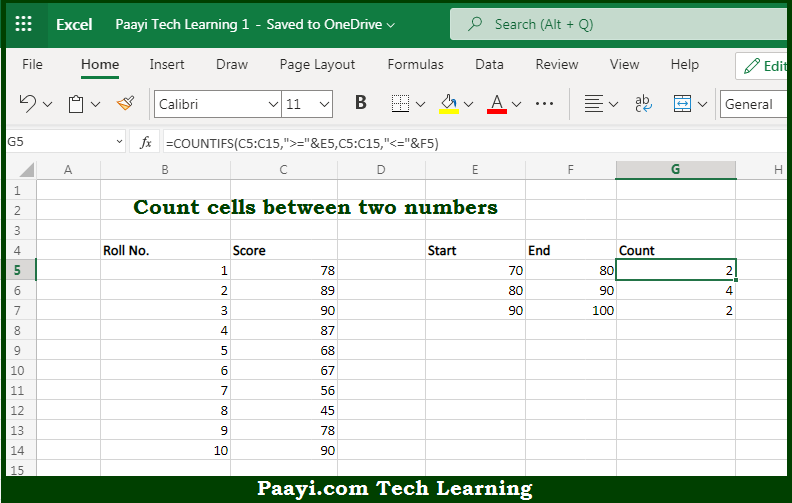# Learn How to Count Cells Between Two Numbers in Microsoft Excel

Written by | 0 Comments | 621 Views

In this article, you will learn how to use the Microsoft Excel COUNT formula and its purpose in Microsoft Excel. You will also get to know how to count Cells Between Two Numbers and see the generic formula.

Count Cells Between Two Numbers Using Microsoft Excel Count Formula

The main purpose of the Microsoft Excel COUNT formula is to count the number of cells that contain values between two numbers in a range of cells. Here we will learn how to count cells between two numbers.  That implies, with the help of the COUNT formula you can able to count the number of cells that contain values between two numbers in a range of cells, you can use the COUNTIFS function. In the generic form of the formula, range represents a range of cells that contain numbers. So, with the help of the COUNT formula, you can able to count the number of cells that contain values between two numbers in a range of cells.

General Syntax of the COUNT Formula To Count Cells Between Two Numbers

=COUNTIFS(range,">="&A1,range,"<"&B1)

The Explanation for the Count Cells Between Two NumbersSo we know that Microsoft Excel COUNT formula you can able to count the number of cells that contain values between two numbers in a range of cells. Here we will learn how to count cells between two numbers. The main purpose of the above example is to count numbers that fall within specific ranges as shown. The lower value comes from the "Start" column, and the upper value comes from the "End" column. For every range, we have included the lower value but excluded the upper value.

The formula used to solve this problem is based on the COUNTIFS function, which is designed to count cells that meet multiple criteria. So, with the help of the COUNT formula, you can able to count the number of cells that contain values between two numbers in a range of cells.